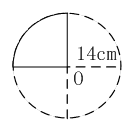### Sample Problem

Find the perimeter of the figure below. (Take π = 22/7)The perimeter of the figure is cm.

#### Solution

The circumference of the circle is:

2 × 22/7 × 14 = 88 cm

So 1/4 of the circumference of the circle is:

88 ÷ 4 = 22 cm

So the perimeter of the figure is:

22 + 14 × 2 = 50 cm